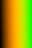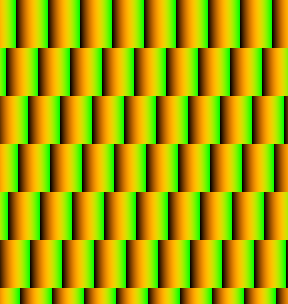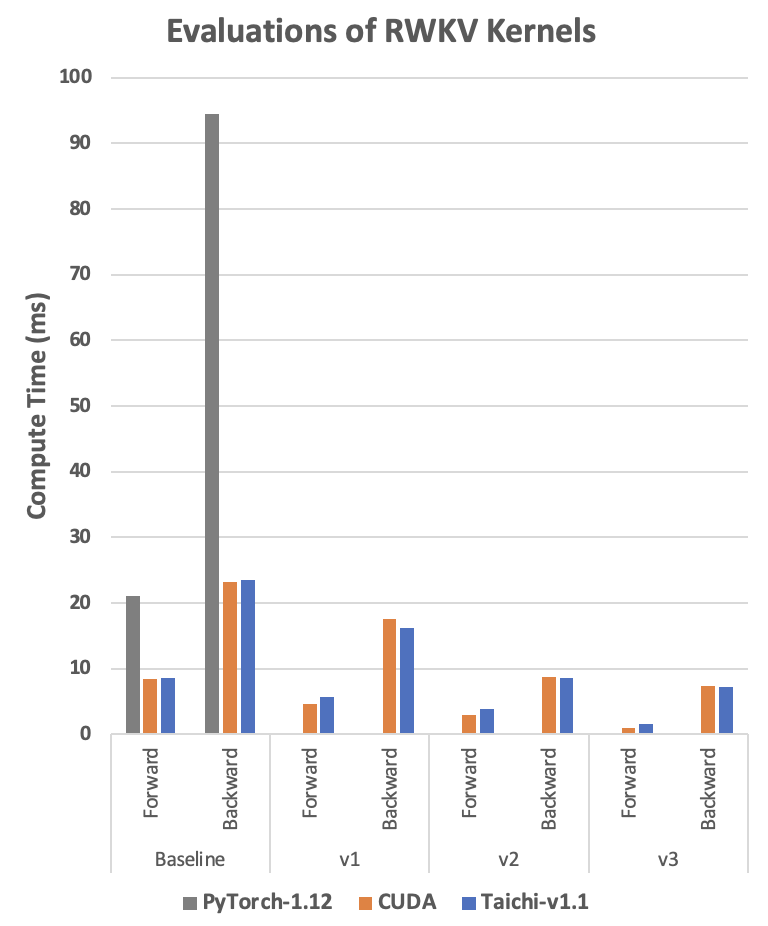Version: v1.6.0

# 用 Taichi 加速 PyTorch

Taichi 和 Torch 的应用场景不同，但可以相互补充。

• Taichi 对并行的控制更精细，且支持颗粒度更高的操作（元素层面），从而给予用户更大的灵活度。
• Torch 将此类细节抽象到 tensor 层面的操作中，好比乐高积木，使用户能够集中精力构建 ML（机器学习）模型。

## 数据预处理

• 使用 Python 或 PyTorch 迭代矩阵元素。
• 编写 C++/CUDA 算子，并通过 Python 定制的算子扩展接入 PyTorch 。

1. 创建一个“砖块”并用渐变色填充。2. 在水平方向重复砖块，采用固定的偏移量形成交错的布局。### 使用 PyTorch 进行边缘填充

``def torch_pad(arr, tile, y):    # image_pixel_to_coord    arr[:, :, 0] = image_height - 1 + ph - arr[:, :, 0]    arr[:, :, 1] -= pw    arr1 = torch.flip(arr, (2, ))    # map_coord    v = torch.floor(arr1[:, :, 1] / tile_height).to(torch.int)    u = torch.floor((arr1[:, :, 0] - v * shift_y) / tile_width).to(torch.int)    uu = torch.stack((u, u), axis=2)    vv = torch.stack((v, v), axis=2)    arr2 = arr1 - uu * shift_x - vv * shift_y    # coord_to_tile_pixel    arr2[:, :, 1] = tile_height - 1 - arr2[:, :, 1]    table = torch.flip(arr2, (2, ))    table = table.view(-1, 2).to(torch.float)    inds = table.mv(y)    gathered = torch.index_select(tile.view(-1), 0, inds.to(torch.long))    return gatheredwith Timer():    gathered = torch_pad(coords, tile, y)    torch.cuda.synchronize(device=device)``

### 使用 Taichi 进行边缘填充

Taichi 自动并行运行顶层循环，且使用 Taichi 编写的矩阵运算可读性更强。 此外，正如以下代码所示，`ti_pad()` 直接接收 PyTorch 的 tensor，因而可以重用分配给 PyTorch 的内存，并且不会因两个框架间的数据传输造成额外的开销。

``@ti.kerneldef ti_pad(image_pixels: ti.types.ndarray(), tile: ti.types.ndarray()):    for row, col in ti.ndrange(image_height, image_width):        # image_pixel_to_coord        x1, y1 = ti.math.ivec2(col - pw, image_height - 1 - row + ph)        # map_coord        v: ti.i32 = ti.floor(y1 / tile_height)        u: ti.i32 = ti.floor((x1 - v * shift_y) / tile_width)        x2, y2 = ti.math.ivec2(x1 - u * shift_x - v * shift_y,                 y1 - u * shift_x - v * shift_y)        # coord_to_tile_pixel        x, y = ti.math.ivec2(tile_height - 1 - y2, x2)        image_pixels[row, col] = tile[x, y]with Timer():    ti_pad(image_pixels, tile）    ti.sync()``

### 性能比较

`torch_pad()` 启动了 58 个 CUDA kernel，而 Taichi 将所有计算都编译成一个 CUDA kernel。 CUDA kernel 越少，产生的 GPU 启动开销就越少。 此外，Taichi kernel 能够比 PyTorch kernel 节省更多冗余的内存操作。 GPU 启动开销和冗余的内存操作是程序优化和加速的潜在来源。

Kernel 函数平均用时（毫秒）启动的 CUDA kernel 数量
`torch_pad()`30.39258
`ti_pad()`0.2671
• GPU：RTX3090
• PyTorch 版本：v1.12.1；Taichi 版本：v1.1.0
• 实际加速比可能根据你的具体实现和 GPU 设置而变化。

## 定制 ML 算子

1. 遍历两个输入的 tensor，即 `w``k`
2. 计算 `w``k` 中对应位置元素的乘积 `s`
3. `s` 保存到输出的 tensor `out` 中。

Python最慢
PyTorch
CUDA
Taichi媲美甚至超过 CUDA

### 使用 Python 实现深度卷积算子

Python 参考代码简单易懂， 但速度太慢，结果甚至难以放进下文的比较图中。

``def run_formula_very_slow(w, k, B, C, T, eps):    out = torch.empty((B, C, T), device='cpu')    for b in range(B):        for c in range(C):            for t in range(T):                s = eps                for u in range(t-T+1, t+1):                    s += w[c][(T-1)-(t-u)] * k[b][c][u+T-1]                out[b][c][t] = s    return out``

### 使用 PyTorch 实现深度卷积算子

``out = eps + F.conv1d(nn.ZeroPad2d((T-1, 0, 0, 0))(k), w.unsqueeze(1), groups=C)``

### 使用 CUDA 实现深度卷积算子

CUDA 参考代码的可读性要低得多：最外层循环的定义隐含在线程并行中。 索引的计算很复杂，每个元素在矩阵中的位置并不一目了然。 而且使用 CUDA 实现更为复杂的算法很容易出错。

``__global__ void kernel_forward(const float* w, const float* k, float* x,                               const float eps, const int B, const int C, const int T){    const int i = blockIdx.y;    const int t = threadIdx.x;    float s = eps;    const float* www = w + (i % C) * T + (T - 1) - t;    const float* kk = k + i * T;    for (int u = 0; u <= t; u++){        s += www[u] * kk[u];    }    x[i * T + t] = s;}``

### 使用 Taichi 实现深度卷积算子

Taichi 参考代码与 Python 版本几乎完全相同。 Taichi 相比于 CUDA 的一个优势在于，你可以轻松用 Taichi 实现相似的性能而无需担心并行和指针偏移等低级细节。

``@ti.kerneldef taichi_forward_v0(        out: ti.types.ndarray(ndim=3),        w: ti.types.ndarray(ndim=3),        k: ti.types.ndarray(ndim=3),        eps: ti.f32):    for b, c, t in out:        s = eps        for u in range(t-T+1, t+1):            s += w[c, 0, (T-1)-(t-u)] * k[b, c, u+T-1]        out[b, c, t] = s``

### 性能比较• 表中的 RWKV 运算时间以毫秒为单位。 运算时间越少，性能越好。
• 基准（Baseline）：参考代码直接实现算法，不做任何改动。
• v1 ～ v3：三种不同的优化版本。

## 总结

PyTorch 能够有效地处理机器学习中的大部分计算任务。 但仍有需求尚未得到解决，比如对许多算子的原生支持和不尽如人意的运行时性能。

• 易读性，
• 优化内存消耗，
• 媲美 CUDA 的运行时性能，
• 易移植性，可鼓励社区分享可复现的代码。